geometryFromEdges

Create 2-D geometry from decomposed geometry matrix

Description

example

geometryFromEdges(model,g) adds the 2-D geometry described in g to the model container.

pg = geometryFromEdges(model,g) additionally returns the geometry to the Workspace.

Examples

collapse all

Create a decomposed solid geometry model and include it in a PDE model.

Create a default scalar PDE model.

model = createpde;

Define a circle in a rectangle, place these in one matrix, and create a set formula that subtracts the circle from the rectangle.

R1 = [3,4,-1,1,1,-1,0.5,0.5,-0.75,-0.75]';
C1 = [1,0.5,-0.25,0.25]';
C1 = [C1;zeros(length(R1) - length(C1),1)];
gm = [R1,C1];
sf = 'R1-C1';

Create the geometry.

ns = char('R1','C1');
ns = ns';
g = decsg(gm,sf,ns);

Include the geometry in the model and plot it.

geometryFromEdges(model,g);
pdegplot(model,'EdgeLabels','on')
axis equal
xlim([-1.1,1.1])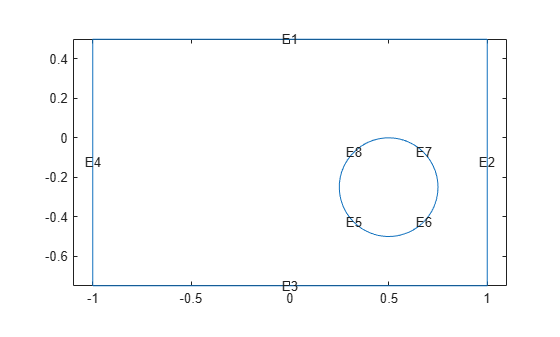The toolbox provides the several geometry functions. Specify them by using the following function handles.

model = createpde;
g = geometryFromEdges(model,@cardg);
pdegplot(model)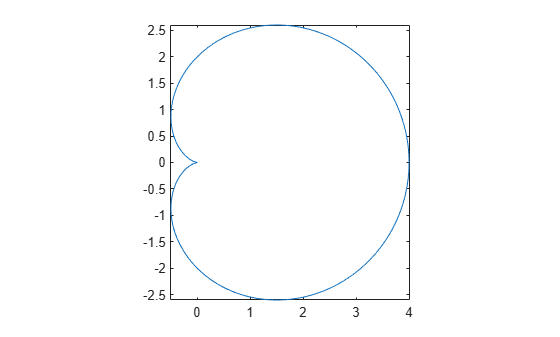clear model
model = createpde;
g = geometryFromEdges(model,@circleg);
pdegplot(model)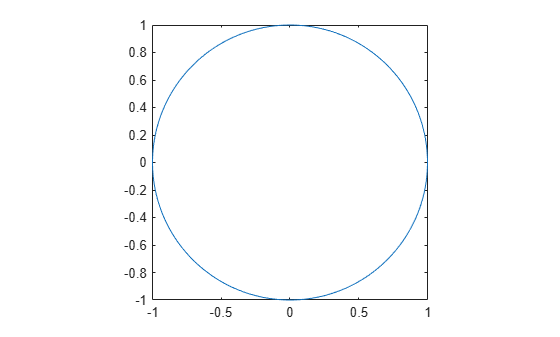clear model
model = createpde;
g = geometryFromEdges(model,@cirsg);
pdegplot(model)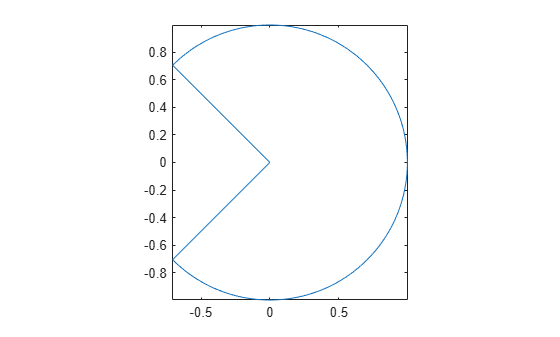clear model
model = createpde;
g = geometryFromEdges(model,@crackg);
pdegplot(model)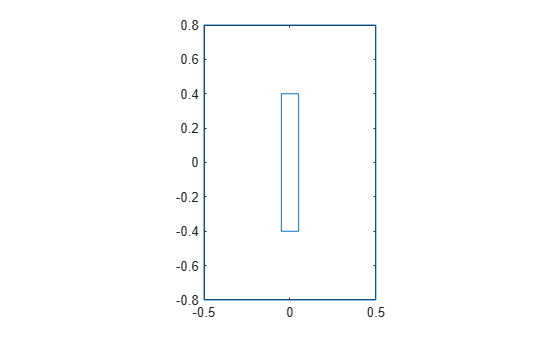clear model
model = createpde;
g = geometryFromEdges(model,@lshapeg);
pdegplot(model)clear model
model = createpde;
g = geometryFromEdges(model,@scatterg);
pdegplot(model)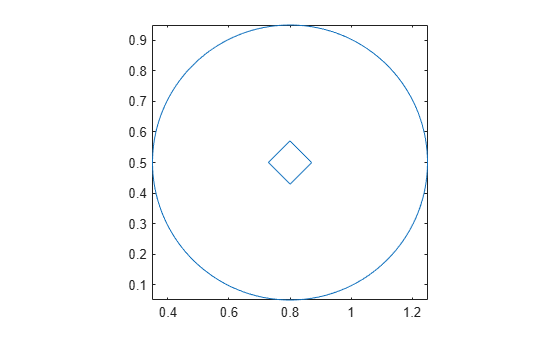clear model
model = createpde;
g = geometryFromEdges(model,@squareg);
pdegplot(model)Input Arguments

collapse all

Model object, specified as a PDEModel object, ThermalModel object, StructuralModel object, or ElectromagneticModel object.

Example: model = createpde(3)

Example: structuralmodel = createpde('structural','static-solid')

Example: emagmodel = createpde('electromagnetic','electrostatic')

Geometry description, specified as a decomposed geometry matrix, as the name of a geometry function, or as a handle to a geometry function. For details about a decomposed geometry matrix, see decsg.

A geometry function must return the same result for the same input arguments in every function call. Thus, it must not contain functions and expressions designed to return a variety of results, such as random number generators.

Example: geometryFromEdges(model,@circleg)

Data Types: double | char | function_handle

Output Arguments

collapse all

Geometry object, returned as an AnalyticGeometry Properties object. This object is stored in model.Geometry.